# Balls

From the urn in which are 7 white balls and 17 red, gradually drag 3-times without replacement.

What is the probability that pulls balls are in order: red red red?

p =  33.6 %

### Step-by-step explanation:

$p=100\mathrm{%}\cdot \frac{17-0}{7+17-0}\cdot \frac{17-1}{7+17-1}\cdot \frac{17-2}{7+17-2}=33.6\mathrm{%}$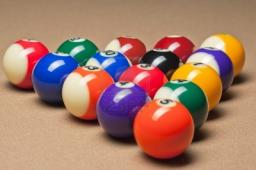Did you find an error or inaccuracy? Feel free to write us. Thank you!Tips to related online calculators
Would you like to compute count of combinations?

## Related math problems and questions:

• Three colors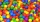Find the probability that 3 balls of the same color will be drawn from fate with 10 white, 10 red, and 10 blue balls.
• White and black ballsThere are 7 white and 3 black balls in an opaque pocket. The balls are the same size. a) Randomly pull out one ball. What is the probability that it will be white? We pull out one ball, see what color it is and return it to the pocket. Then we pull out th
• BallsThe urn is 8 white and 6 black balls. We pull 4 randomly balls. What is the probability that among them will be two white?
• Round destiny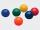There are 5 white and 10 red balls in the destiny. 4 balls will be drawn at random. What is the probability of the event "at least 2 spheres are white"?
• Components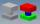In the box are 8 white, 4 blue and 2 red components. What is the probability that we pull one white, one blue and one red component without returning?
• Balls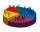We have n identical balls (numbered 1-n) is selected without replacement. Determine 1) The probability that at least one tensile strength number coincides with the number of balls? 2) Determine the mean and variance of the number of balls, which coincides
• Red ballsIn the bag there are 3 red, 12 blue and 8 green balls. How many red balls we must be attached to the bag if we want the probability of pulling out the red balls was 20%?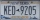A license plate has 3 letters followed by 4 numbers. Repeats are not allowed for the letters, but they are for the numbers. If they are issued at random, what is the probability that the 3 letters are in alphabetical order and the 3 numbers are consecutiv
• Green - Red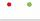We have 5 bags. Each consist one green and 2 red balls. From each we pull just one ball. What is the probability that we doesn't pull any green ball?
• White balls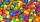I have 3 white balls and 5 red balls in my pocket. How many least balls do I have to remove from the bag to make sure that the pulled out ball is definitely red?
• Tokens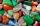In the non-transparent bags are red, white, yellow, blue tokens. We 3times pull one tokens and again returned it, write down all possibilities.
• One greenIn the container are 45 white and 15 balls. We randomly select 5 balls. What is the probability that it will be a maximum one green?
• A boxA box contains 6 red balls, 5 blue, and 4 white balls. Find the probability of drawing a) red ball b) non-white ball.
• Events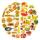Event P has probability of 0.84. What is the probability that the event P occurs in 3, 5, 7 try.
• Three reds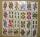What is the probability that when choosing 3 carats from seven carats, all 3 reds will be red?
• CardsSuppose that are three cards in the hats. One is red on both sides, one of which is black on both sides, and a third one side red and the second black. We are pulled out of a hat randomly one card and we see that one side of it is red. What is the probabi
• Hearts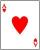5 cards are chosen from a standard deck of 52 playing cards (13 hearts) with replacement. What is the probability of choosing 5 hearts in a row?